# Pair of straight lines intermediate mathematics first year chapter 4 solutions

Mathematics intermediate 1a and 1b solutions  for some problems are available here. These are very simple to understand.

Inter first year 1a : Functions, mathematical inductions, addition of vectors, product of vectors and trigonometry.

Inter first year 1b : Locus, transformation of axes, the straight line, pair of straight lines, three dimensional coordinates, direction cosines & ratios and applications of derivatives.

You can see the solutions for junior inter maths 1b

1. Locus

12. Rate measure

You can also see the solutions for junior inter maths 1A

3. Matrices

## Coordinate geometry – pair of straight lines inter maths 1b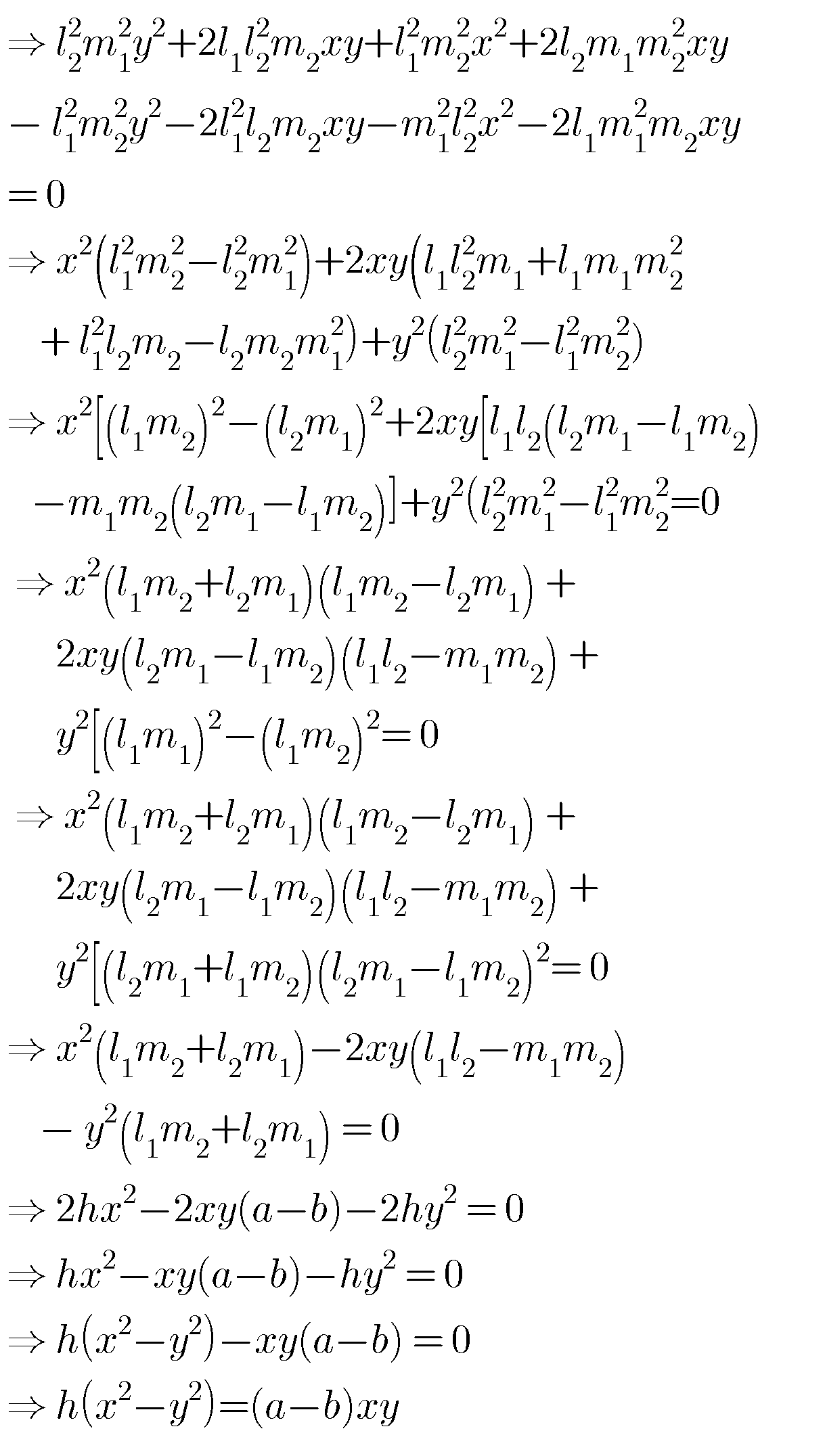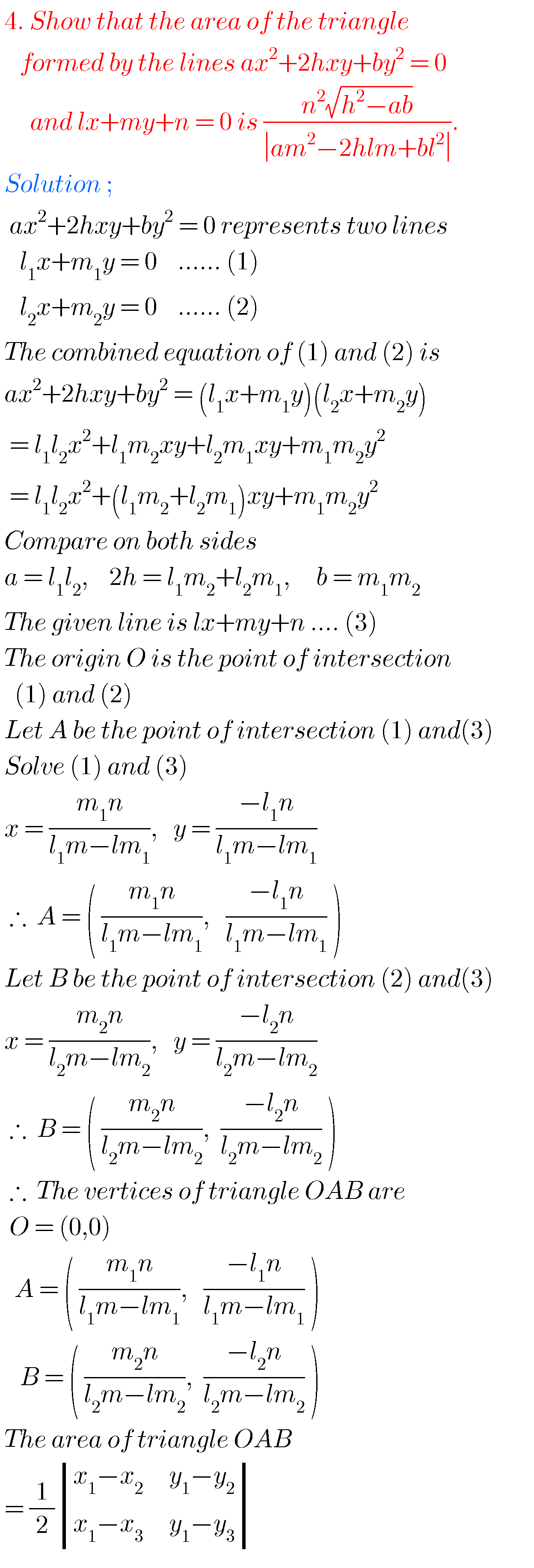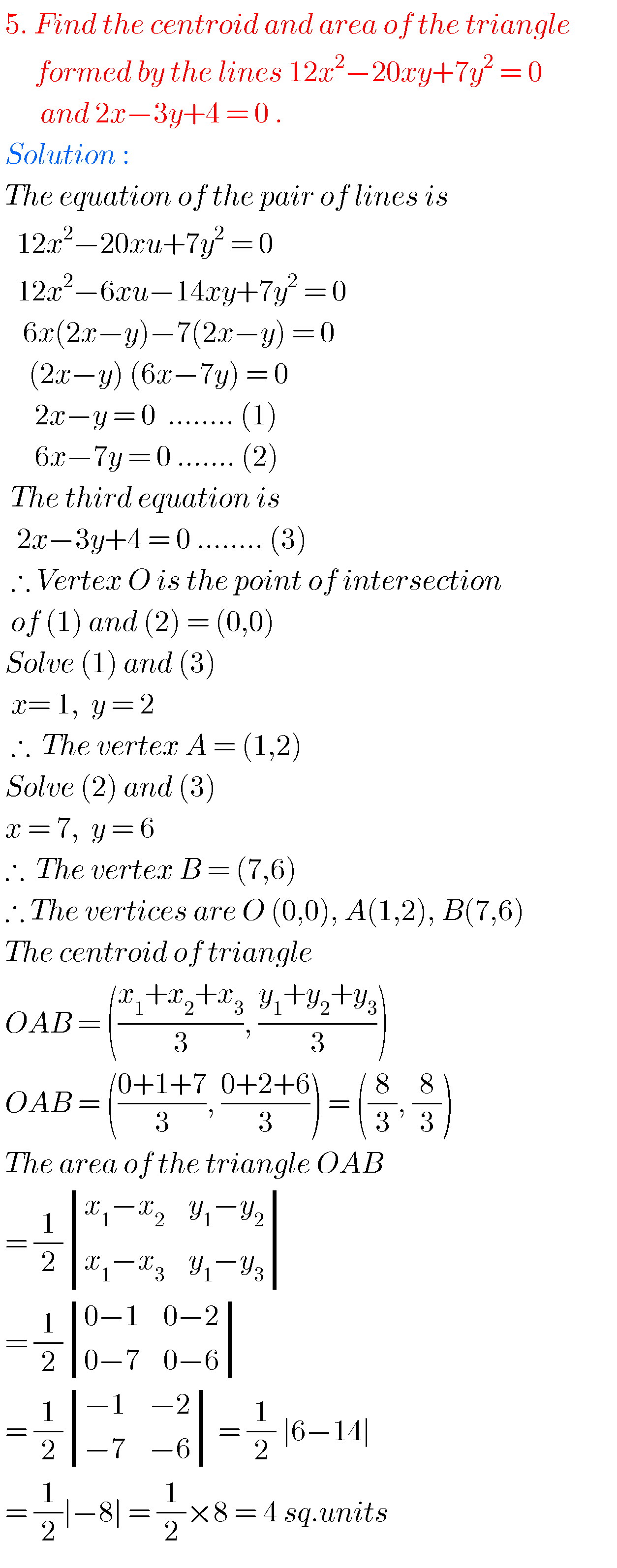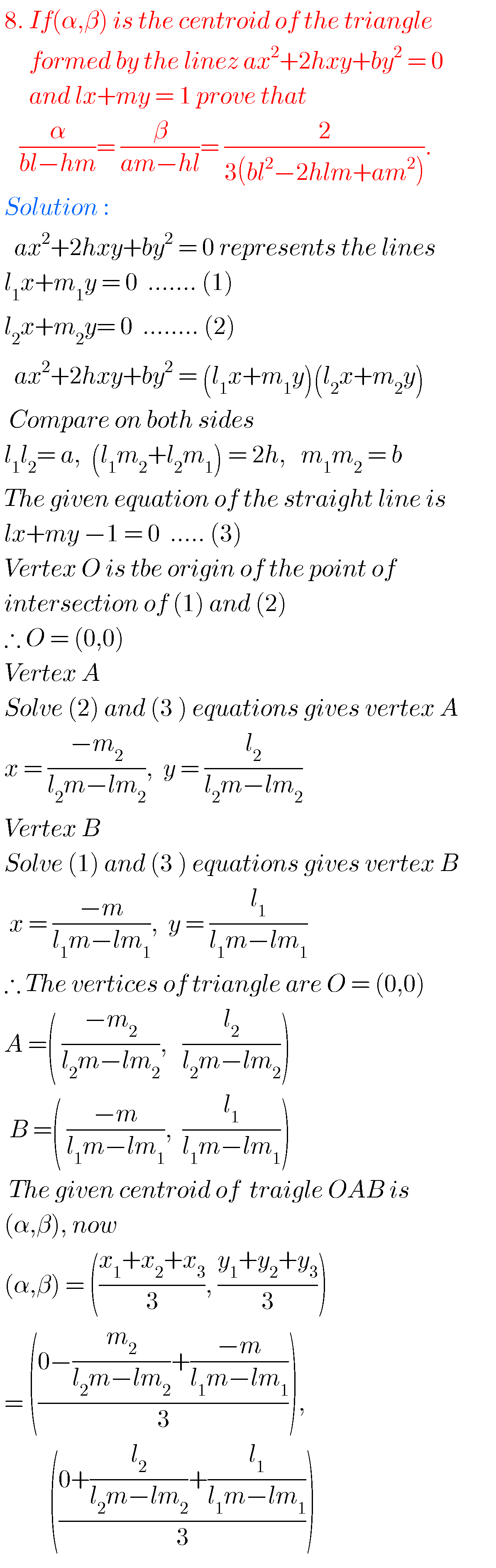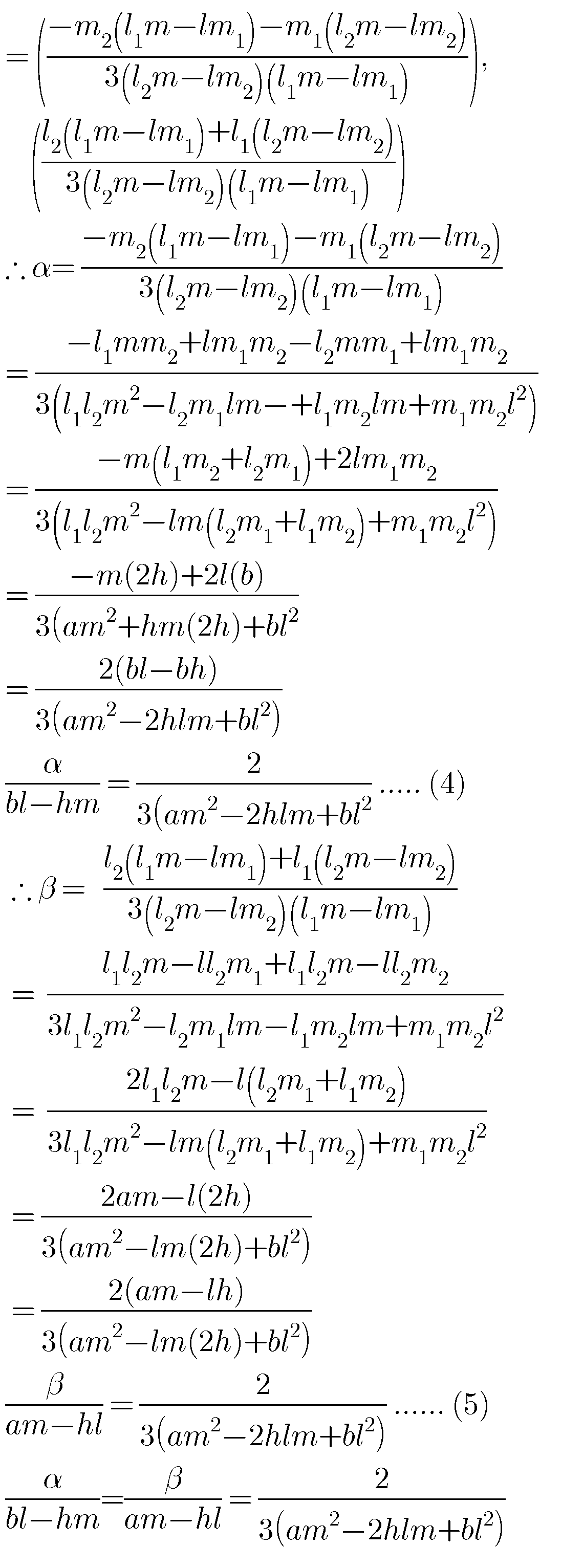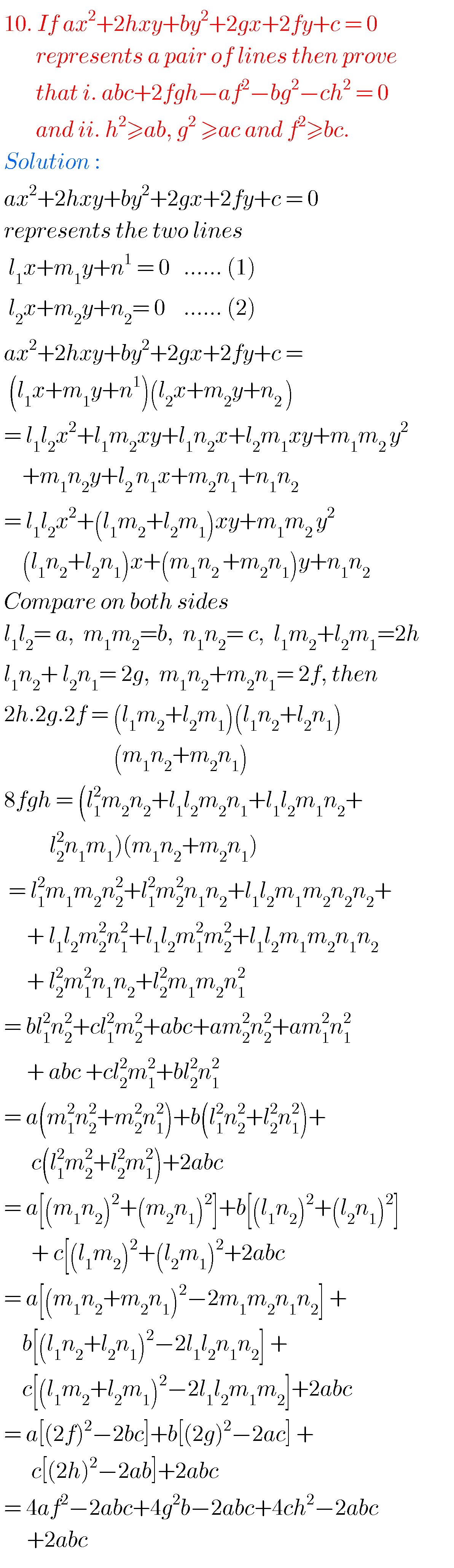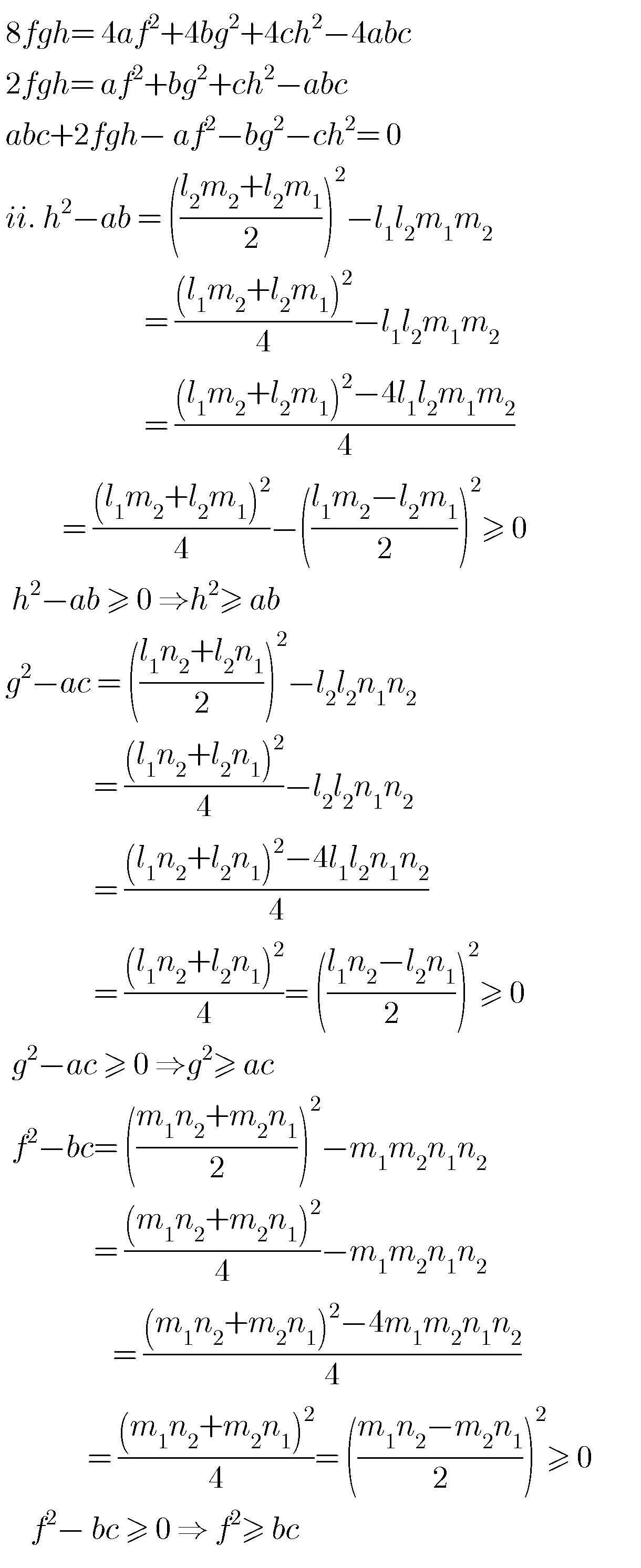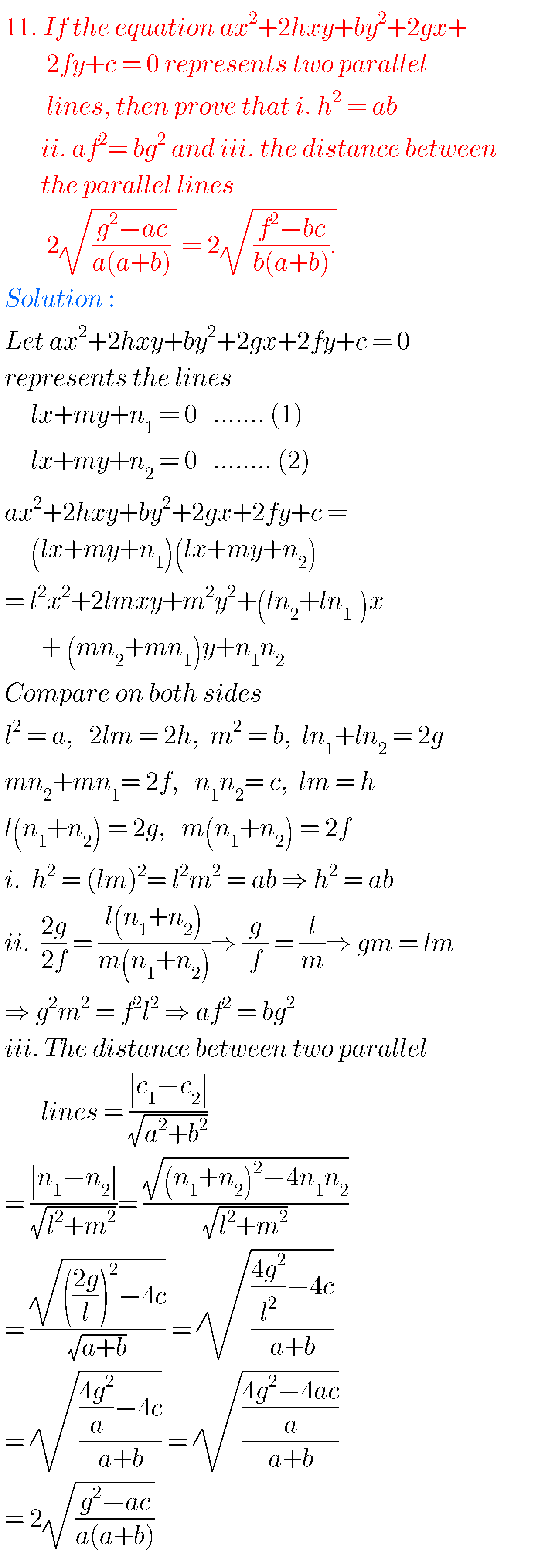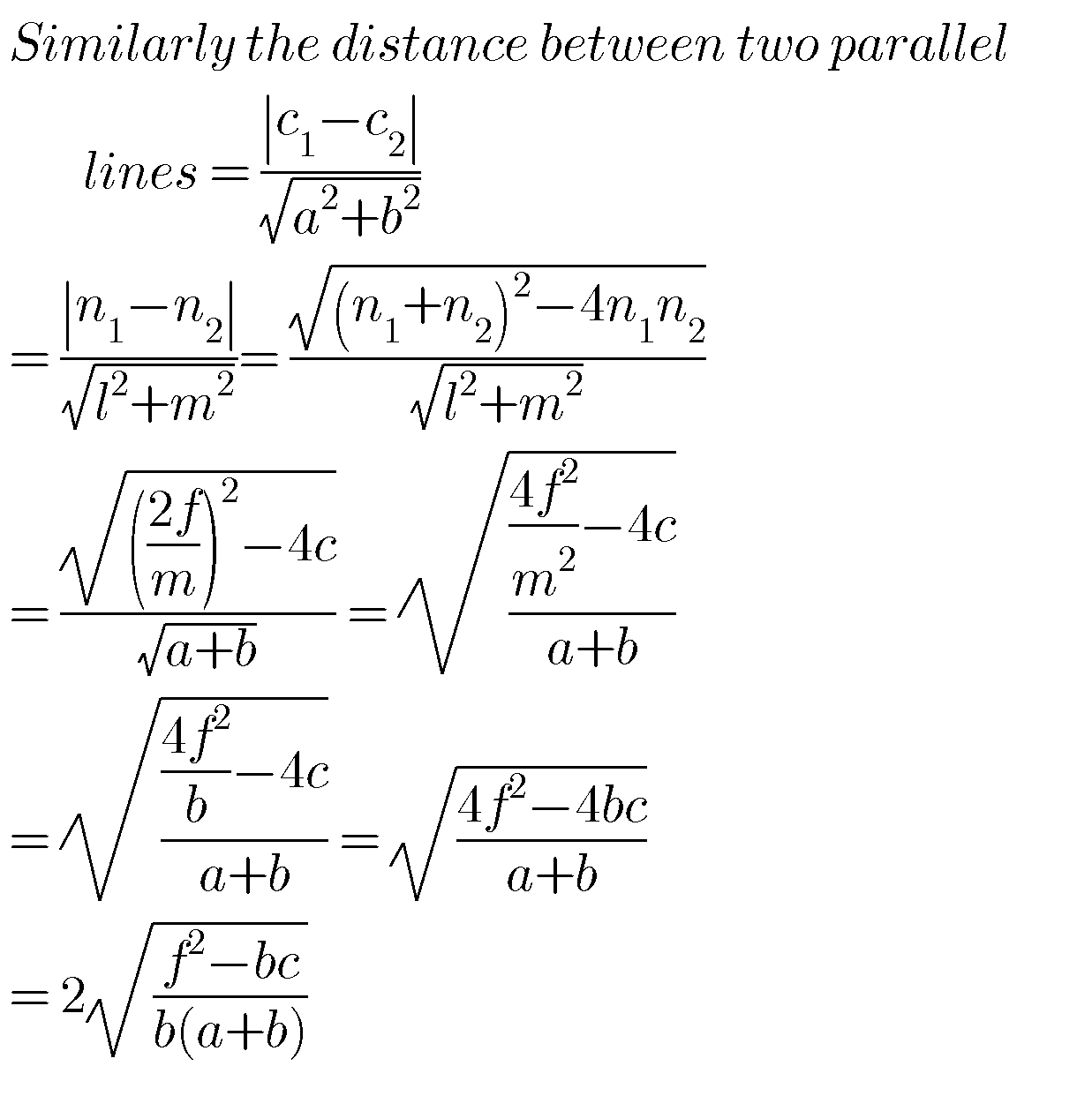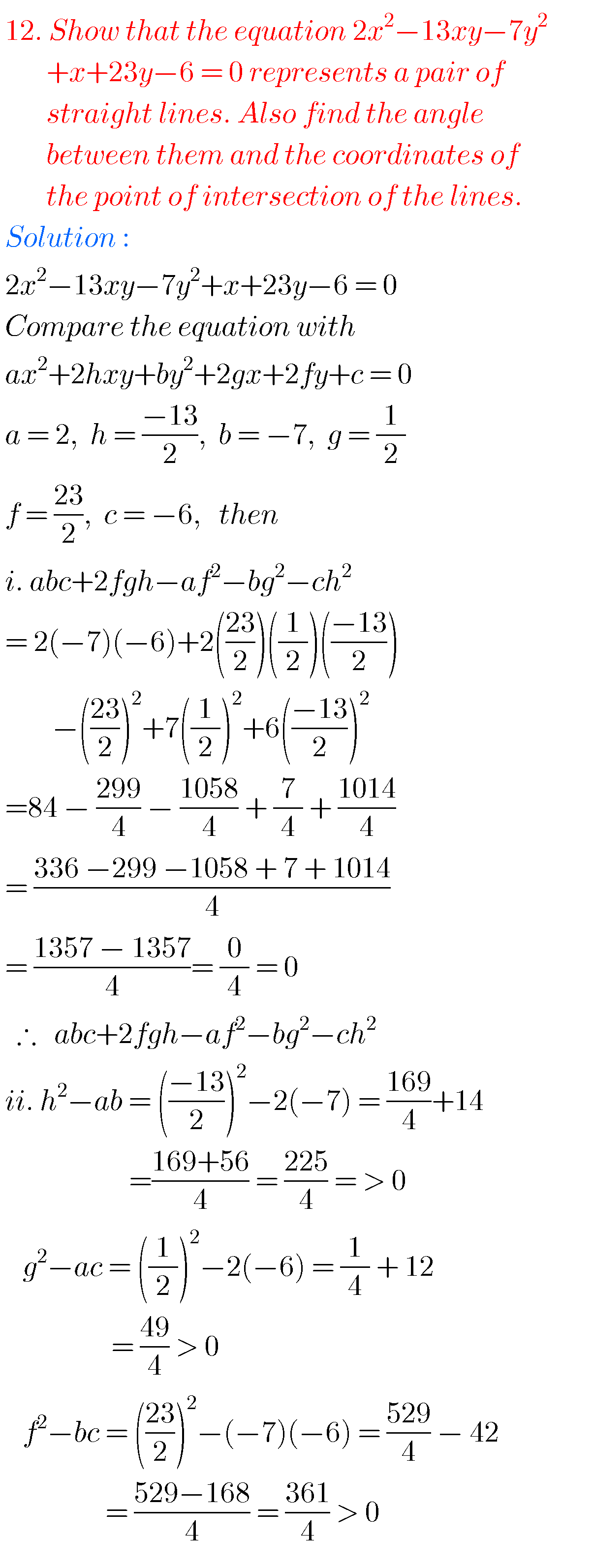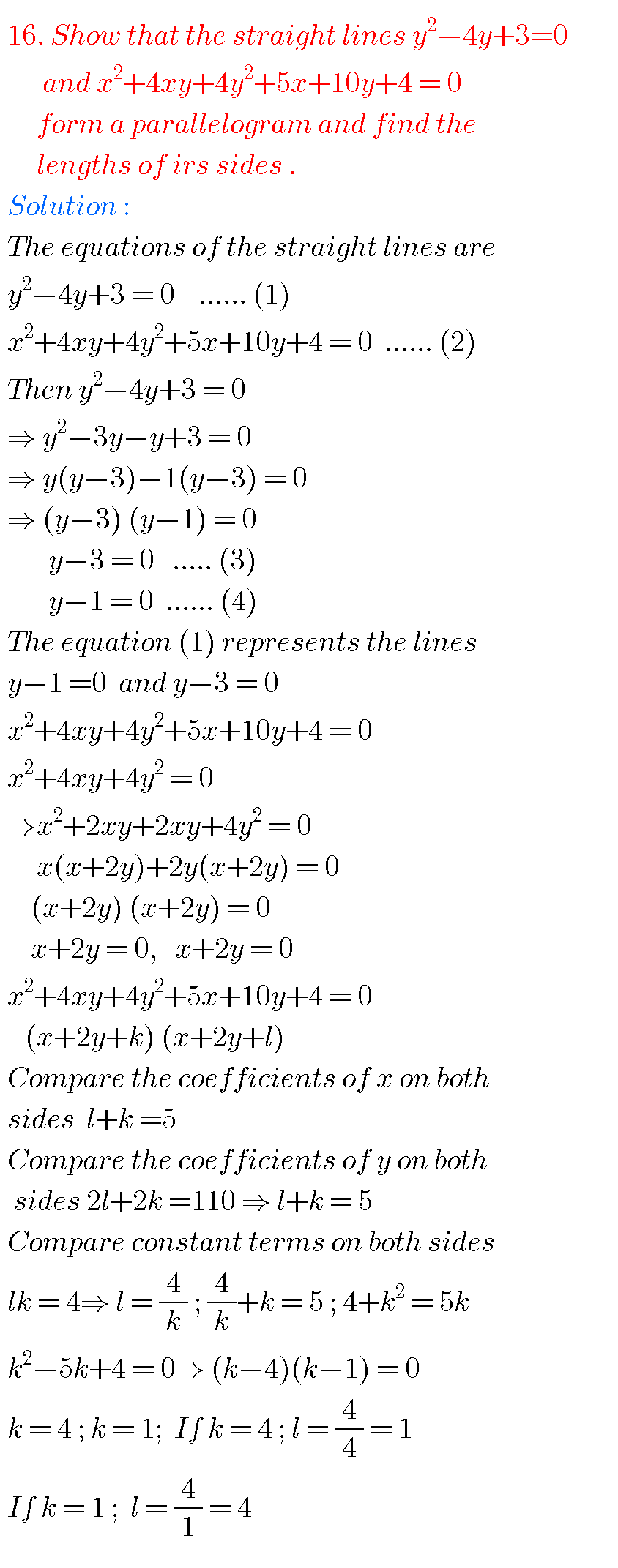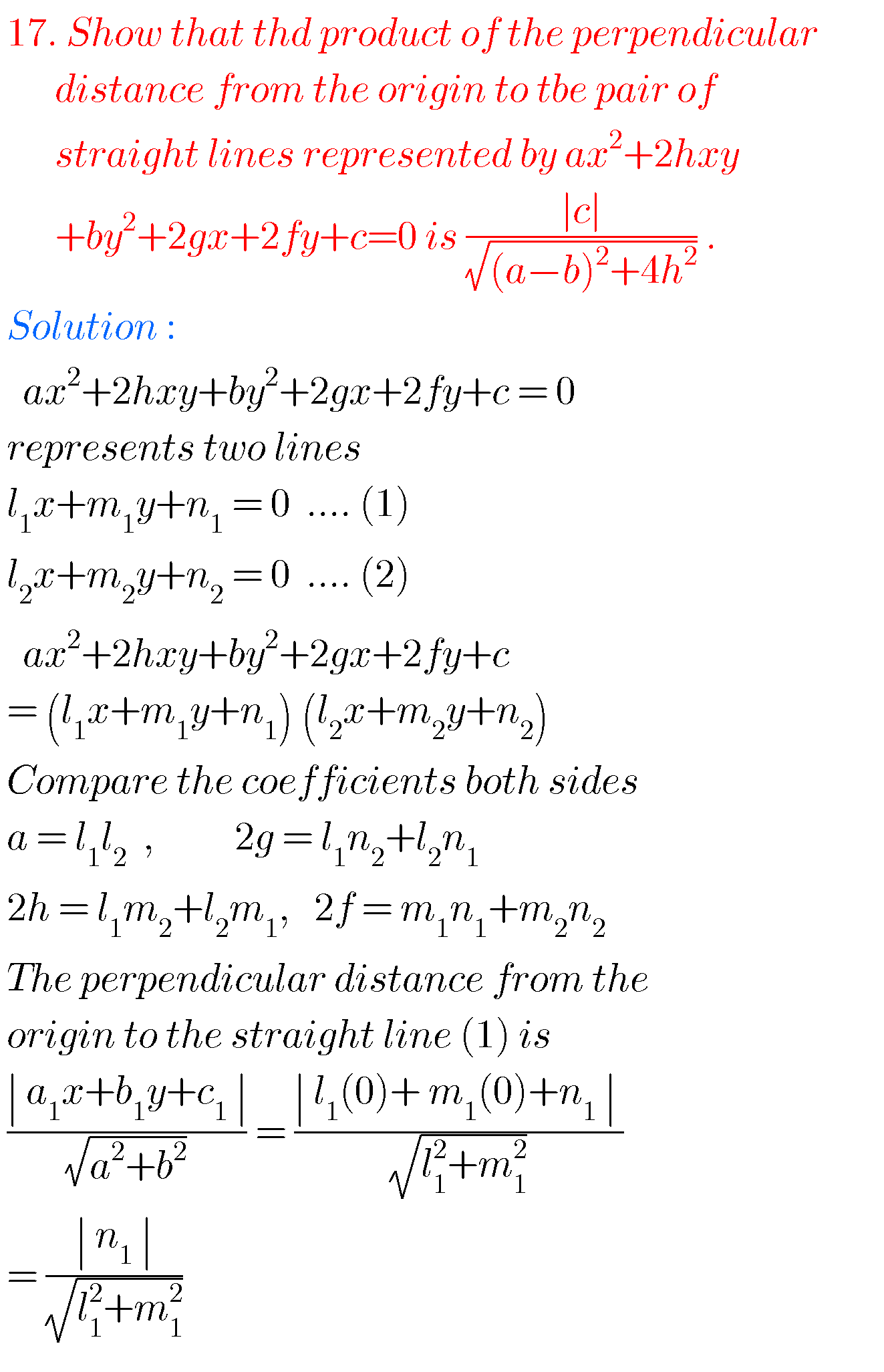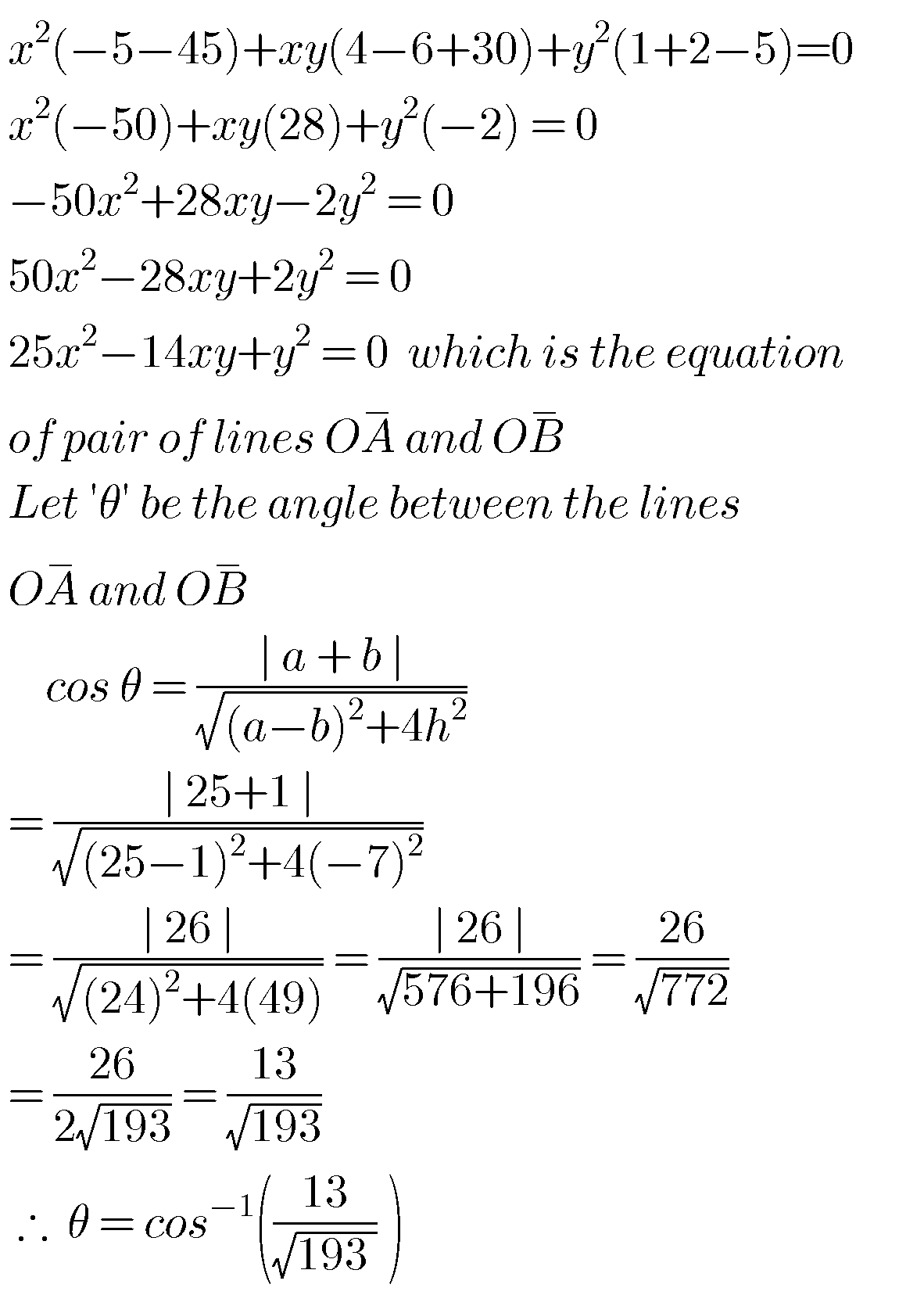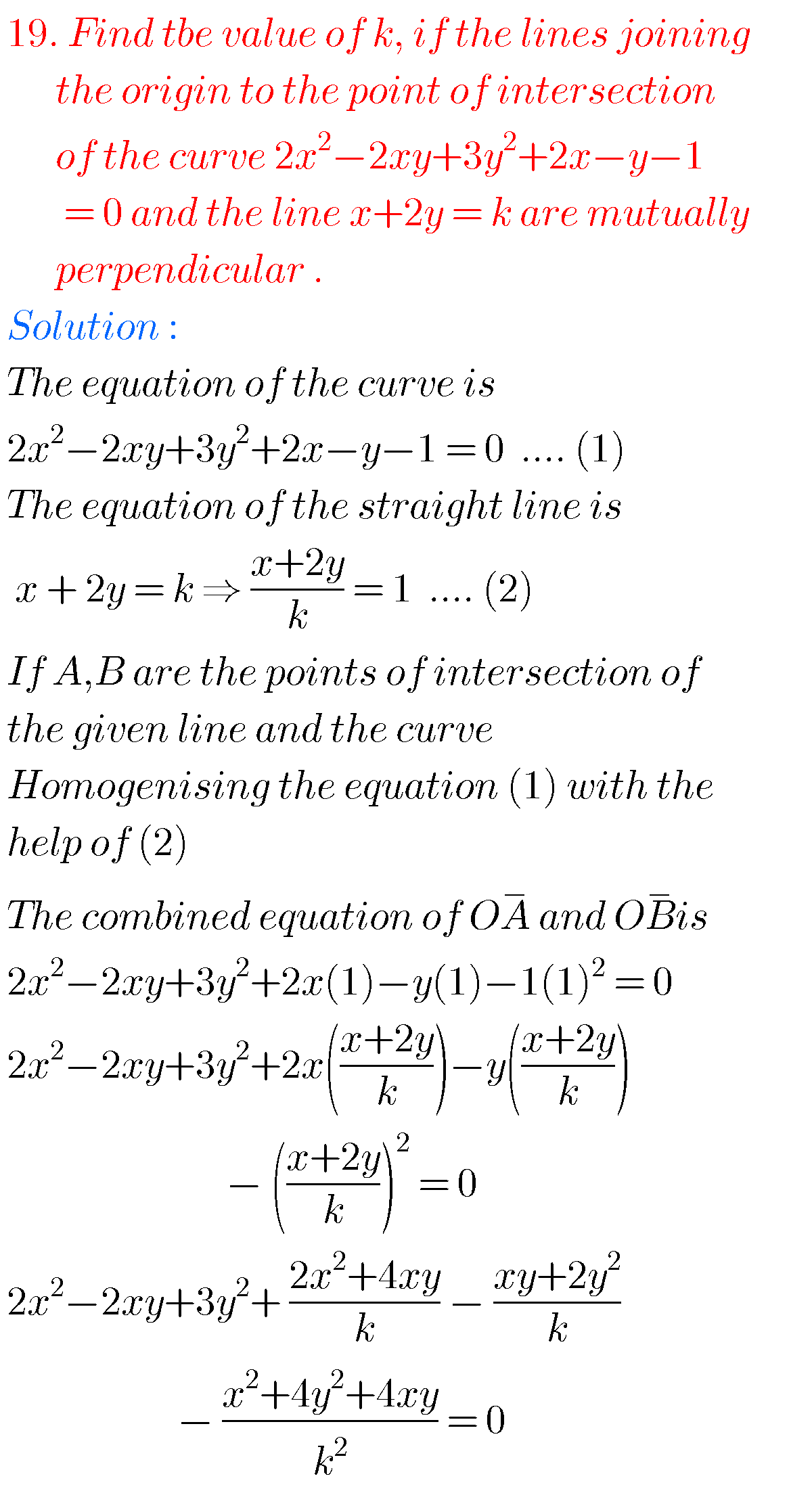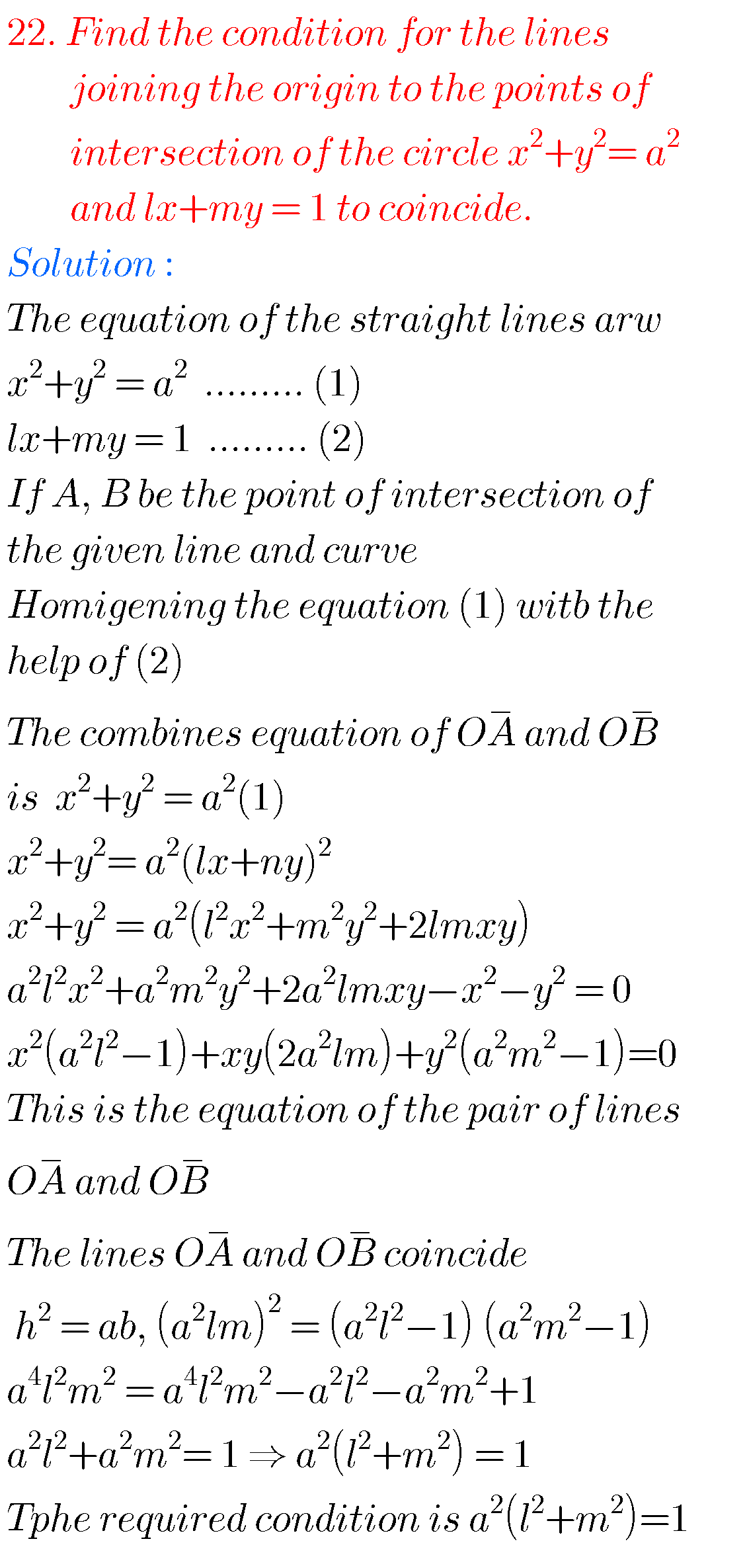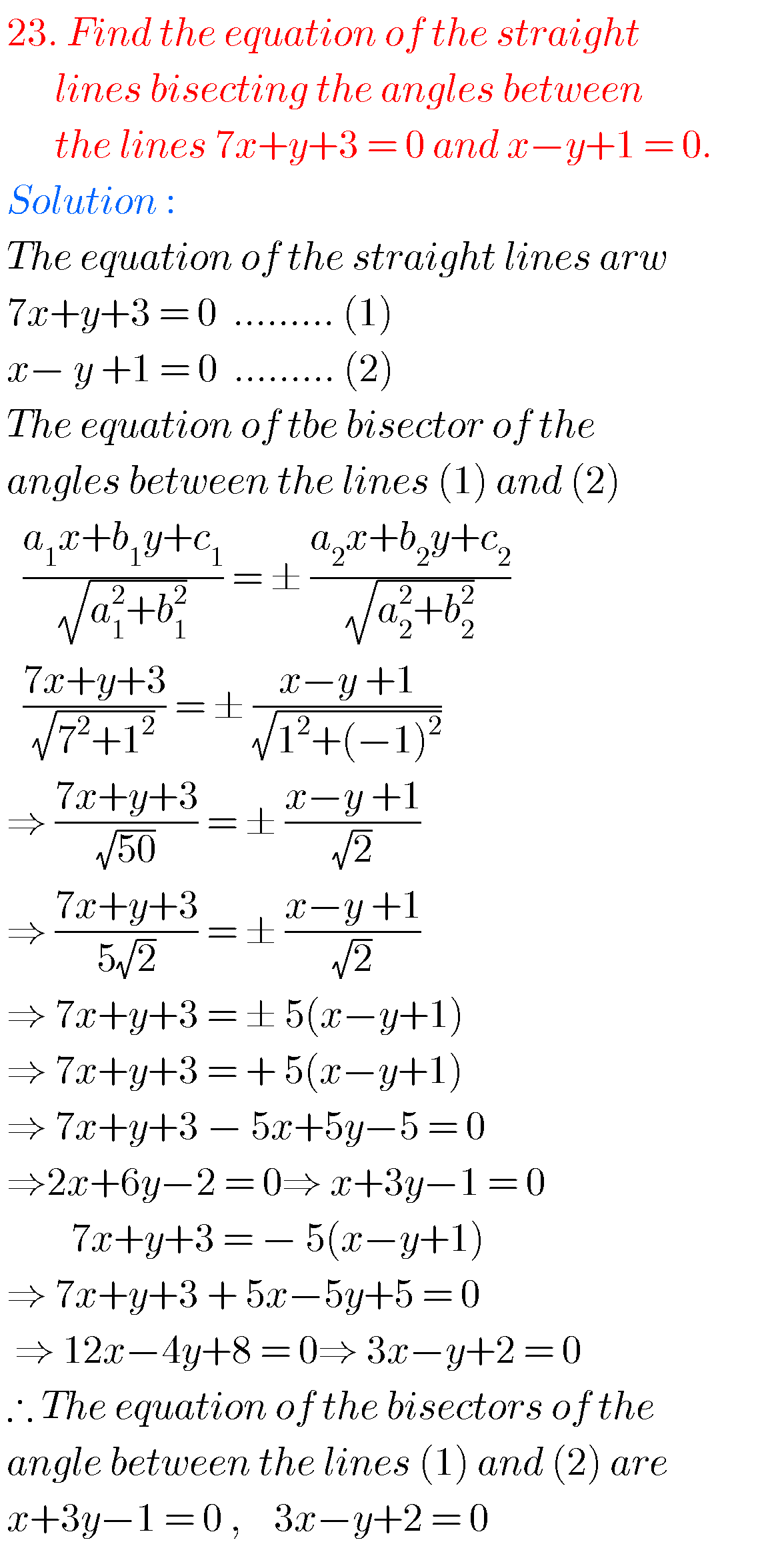Note – Observe the solutions and try them in your own methods.

Inter maths 1a solutions

Inter maths trigonometry solutions